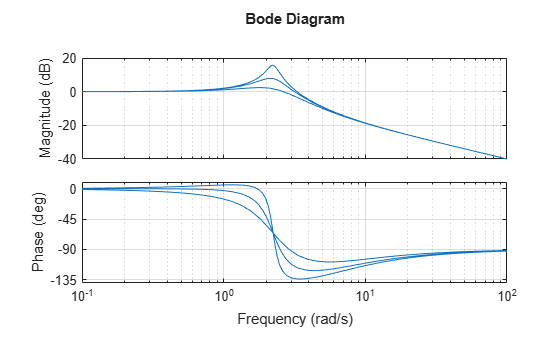# Accessing and Modifying the Model Data

This example shows how to access or edit parameter values and metadata in LTI objects.

### Accessing Data

The `tf`, `zpk`, `ss`, and `frd` commands create LTI objects that store model data in a single MATLAB® variable. This data includes model-specific parameters (e.g., A,B,C,D matrices for state-space models) as well as generic metadata such as input and output names. The data is arranged into a fixed set of data fields called properties.

You can access model data in the following ways:

• The `get` command

• Structure-like dot notation

• Data retrieval commands

For illustration purposes, create the SISO transfer function (TF):

`G = tf([1 2],[1 3 10],'inputdelay',3)`
```G = s + 2 exp(-3*s) * -------------- s^2 + 3 s + 10 Continuous-time transfer function. ```

To see all properties of the TF object `G`, type

`get(G)`
``` Numerator: {[0 1 2]} Denominator: {[1 3 10]} Variable: 's' IODelay: 0 InputDelay: 3 OutputDelay: 0 InputName: {''} InputUnit: {''} InputGroup: [1x1 struct] OutputName: {''} OutputUnit: {''} OutputGroup: [1x1 struct] Notes: [0x1 string] UserData: [] Name: '' Ts: 0 TimeUnit: 'seconds' SamplingGrid: [1x1 struct] ```

The first four properties `Numerator`, `Denominator`, `IODelay`, and `Variable` are specific to the TF representation. The remaining properties are common to all LTI representations. You can use `help tf.Numerator` to get more information on the `Numerator` property and similarly for the other properties.

To retrieve the value of a particular property, use

`G.InputDelay % get input delay value`
```ans = 3 ```

You can use abbreviations for property names as long as they are unambiguous, for example:

`G.iod % get transport delay value`
```ans = 0 ```
`G.var % get variable`
```ans = 's' ```

### Quick Data Retrieval

You can also retrieve all model parameters at once using `tfdata`, `zpkdata`, `ssdata`, or `frdata`. For example:

`[Numerator,Denominator,Ts] = tfdata(G)`
```Numerator = 1x1 cell array {[0 1 2]} ```
```Denominator = 1x1 cell array {[1 3 10]} ```
```Ts = 0 ```

Note that the numerator and denominator are returned as cell arrays. This is consistent with the MIMO case where `Numerator` and `Denominator` contain cell arrays of numerator and denominator polynomials (with one entry per I/O pair). For SISO transfer functions, you can return the numerator and denominator data as vectors by using a flag, for example:

`[Numerator,Denominator] = tfdata(G,'v')`
```Numerator = 1×3 0 1 2 ```
```Denominator = 1×3 1 3 10 ```

### Editing Data

You can modify the data stored in LTI objects by editing the corresponding property values with `set` or dot notation. For example, for the transfer function `G` created above,

`G.Ts = 1;`

changes the sample time from 0 to 1, which redefines the model as discrete:

`G`
```G = z + 2 z^(-3) * -------------- z^2 + 3 z + 10 Sample time: 1 seconds Discrete-time transfer function. ```

The `set` command is equivalent to dot assignment, but also lets you set multiple properties at once:

```G.Ts = 0.1; G.Variable = 'q'; G```
```G = q + 2 q^(-3) * -------------- q^2 + 3 q + 10 Sample time: 0.1 seconds Discrete-time transfer function. ```

### Sensitivity Analysis Example

Using model editing together with LTI array support, you can easily investigate sensitivity to parameter variations. For example, consider the second-order transfer function

`$H\left(s\right)=\frac{s+5}{{s}^{2}+2\zeta s+5}$`

You can investigate the effect of the damping parameter `zeta` on the frequency response by creating three models with different `zeta` values and comparing their Bode responses:

```s = tf('s'); % Create 3 transfer functions with Numerator = s+5 and Denominator = 1 H = repsys(s+5,[1 1 3]); % Specify denominators using 3 different zeta values zeta = [1 .5 .2]; for k = 1:3 H(:,:,k).Denominator = [1 2*zeta(k) 5]; % zeta(k) -> k-th model end % Plot Bode response bode(H) grid```